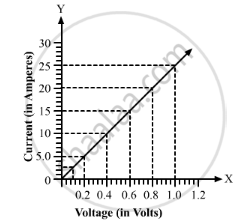Share

# The Values of Potential Difference V Applies Across a Resistor and the Corresponding Values of Current I Flowing in the Resistor Are Given Below: - CBSE Class 10 - Science

ConceptElectric Potential Difference

#### Question

The values of potential difference V applies across a resistor and the corresponding values of current I flowing in the resistor are given below:

 Potential differences, V (in volts) : 2.5 5 10 15 20 25 Current, I (in amperes) : 0.1 0.2 0.4 0.6 0.8 1

#### Solution

From the graph, resistance, R =R=V/I=5/0.2=25ΩIs there an error in this question or solution?

#### Video TutorialsVIEW ALL 

Solution The Values of Potential Difference V Applies Across a Resistor and the Corresponding Values of Current I Flowing in the Resistor Are Given Below: Concept: Electric Potential Difference.
S